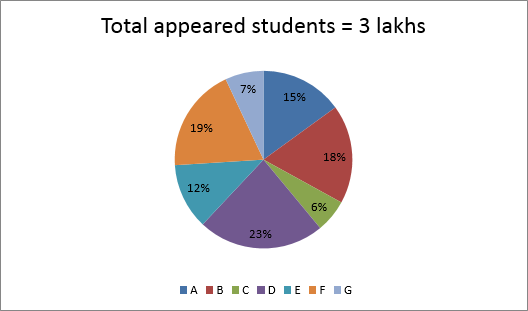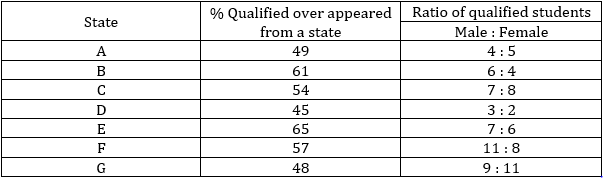### Quantitative Aptitude Quiz For NIACL AO

Numerical Ability or Quantitative Aptitude Section has given heebie-jeebies to the aspirants when they appear for a banking examination. As the level of every other section is only getting complex and convoluted, there is no doubt that this section, too, makes your blood run cold. The questions asked in this section are calculative and very time-consuming. But once dealt with proper strategy, speed, and accuracy, this section can get you the maximum marks in the examination. Following is the Quantitative Aptitude quiz to help you practice with the best of latest pattern questions.

Directions (1-5): Study the following graph and table carefully to answer the questions that follow.

Percentage distribution of students appeared in a competitive exam from seven statesTable shows percentage of students qualified from each state and ratio of male and female qualified studentsQ1. What is the total number of candidates disqualified from state E, B and G together ?
40570
44380
45480
44580
42867
Solution:
Total disqualified students = [35×12+39×18+52×7]×30 = 44580

Q2. What is the total number of female candidates qualified from states F and C together ?
16864
18864
18684
21848
14987
Solution:
Total qualified females = [8/19×57/100×19/100+8/15×54/100×6/100]×3 lakh
= 18864

Q3. Which of the following pair of states have equal number of qualified male candidates ?
B and F
C and G
C and E
A and E
None of these
Solution:
Qualified males in A = 4/9×49/100×15/100×3 lakh = 9800
Qualified males in B = 6/10×61/100×18/100×3 lakh = 19764
Qualified males in C = 7/15×54/100×6/100×3 lakh = 4536
Qualified males in E = 7/13×65/100×12/100×3 lakh = 12600
Qualified males in F = 11/19×57/100×19/100×3 lakh = 18810
Qualified males in G = 9/20×48/100×7/100×3 lakh = 4536

Q4. Find the difference between qualified males from E and C together and qualified females from B and D together?
8640
8460
8200
8160
8920
Solution:
Qualified male from E and C together = 12600 + 4536 = 17136
Qualified females from F and D together
= (4/10×61/100×18/100+2/5×45/100×23/100)×3,00,000 = 25596
∴ Required difference = 8460

Q5. Qualified females from A are what percent (approximate) of appeared candidates from B?
21%
25%
27%
18%
23%

Q6. 15 women can do a piece of work in 12 days while 16 men can do the same work in 10 days. All men and all women work alternately starting with all men, then find the time taken by them to complete the whole work?
10⅓ days
10⅚ days
10⅔ days
12½ days
14 days
Solution:
Let efficiency of every man and every woman be m unit/day and w unit/day respectively 15×12×w=10×16×m
⇒m/w=9/8
Total work=15×12×8=1440 units
In 2 days, total work done=16×9+15×8=264 units
So, in 10 days work done will be=264×5=1320
units Remaining work will be done in = (1440-1320)/(16×9)=5/6
days Total days=10⅚ days

Q7. A train can cross a platform, a tunnel and a pole in 36 sec, 24 sec and 12 sec respectively. Another train whose speed is 72km/hr can cross a pole in 8 sec. If speed of former train is four-fifth of the speed of the latter train, then find sum of length of platform and of tunnel.
476 m
676 m
576 m
756 m
572 m

Q8. Average marks of a student in five subjects is 80. If the average marks of first two subject is 84.5 and the average marks of last who subject is 83. Find the marks of third subject ?
51
53
65
63
60
Solution:
Total marks → 80 × 5 ⇒ 400
Total marks of Ist two subject = 84.5 × 2 = 179
Total marks of last two subject = 83 × 2 = 166
Marks of third subject = 400 – (166 + 169) = 65

Q9. A man can row the boat in downstream from point P to R in 16 hours. But he return from point Q, which is exactly mid-point of P and R in upstream in 12 hours. Find the ratio of speed of stream to the speed of man in still water ?
3 : 5
2 : 3
3 : 2
4 : 3
5 : 1
Solution:
Let speed of man in still water = x km/hr
Speed of current = y km/hr
Let PQ = QR = M km and PR = 2M km
ATQ—
x+y = 2M/16 …(i)
x–y = M/12 …(ii)
on solving (i) and (ii)
x/y = 5/1

Q10. Vaibhav, while buying from wholesaler, gets 17 items at the cost of 10 items. If he allows two successive discounts of 5.88% and ‘a%’ and earns an overall profit of 20%. Then find value of ‘a’.
20
25
35
30
15
Solution:
Let the cost price of 1 item be Rupee 1
and, he bought 17 items
then, cost price for him = 10 × 1 = Rs. 10
ATQ,
10×120/100 = 17×16/17×(100–a)/100
or, 12/16 = (100–a)/100
or, a = 25

Directions (11-15): In each of these questions, two equations (I) and (II) are given. You have to solve both the equations and give answer

Q11. I. 49x² + 84x – 64 = 0
II. 25y² - 100y + 64 = 0
if x>y
if x≥y
if x
if x ≤y
if x = y or no relation can be established between x and y

Q12. I. 4x² - 33x + 63 = 0
II. 10y² - 113y + 318 = 0
if x>y
if x≥y
if x
if x ≤y
if x = y or no relation can be established between x and y

Q13. I. 3x + 4y = 24
II. 2x + 3y = 17
if x>y
if x≥y
if x
if x ≤y
if x = y or no relation can be established between x and y

Q14.if x>y
if x≥y
if x
if x ≤y
if x = y or no relation can be established between x and y

Q15. I. x² – 15x + 56 = 0
II. y² – 13y + 42 = 0
if x>y
if x≥y
if x
if x ≤y
if x = y or no relation can be established between x and y